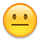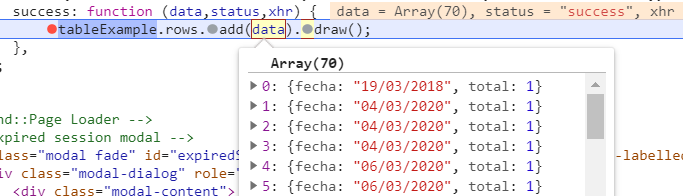# Problem with insert a new row

Hi.

I have a problem when I want to insert data after an ajax call with the JSON of my project.

The definition of my table is:

``````<table class="table table-bordered m-table" id="tableExample">
<tr>
<th class="text-center">Name</th>
<th class="text-center">Created</th>
</tr>
</table>
``````
``````var tableExample = \$('#tableExample').DataTable();
``````
``````\$.ajax({
url: "{{url('/')}}/getusers",
method: "GET",
xhrFields: {
withCredentials: true
},
data: {"CreationDate": Date},
success: function (data,status,xhr) {
\$.each(data, function(index,value){
"Name": value.name,
"Created": value.created
}).draw();
});
},
});
``````

And the error that I get in my screen is:

I debug with Chrome console and data is an array and have the attr "name" and "created".

So, I don't know where could be the errorIf you need some extra info, tell me.

• I'm assuming this is your Datatables initialization:

``````var tableExample = \$('#tableExample').DataTable();
``````

You haven't defined `columns.data` which is needed when adding objects like this:

``````            tableExample.row.add({
"Name": value.name,
"Created": value.created
}).draw();
``````

Without defining `columns.data` Datatables expects the data to be an array for each row instead of the object you are adding. That is basically what the error link http://datatables.net/tn/4 is trying to say.

Looks like you `data` in the `success` function is an array of objects. If so you can just use `tableExample.rows.add( data ).draw();`. Notice the plural `rows`. However if you choose to loop through the data and use `row.add()` remove the chained `.draw()` and use `tableExample.draw();` after the each loop. It will be more efficient to execute `draw()` once rather than for each row added.

Kevin

Yes Kevin, the success is an array of object, look the image.But the error continues• edited June 30 Answer ✓

Looks like the objects and the array have the keys `fecha` and `total`. But you are using something different `value.name` and `value.created`:

``````tableExample.row.add({
"Name": value.name,
"Created": value.created
}).draw();
``````

But the error continues

What did you change? If you added `columns.data` you need to use `fecha` and `total`, like this:

``````columns: [
{data: 'fecha'},
{data: 'total'}
],
``````

Kevin